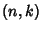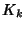## Turán Graph

The-Turán graph is the Extremal Graph onVertices which contains no-Clique. In other words, the Turán graph has the maximum possible number of Edges of any-vertex graph not containing a Complete Graph. Turán's Theorem gives the maximum number of edgesfor the-Turán graph. For,so the Turán graph is given by the Complete Bipartite GraphsAigner, M. Turán's Graph Theorem.'' Amer. Math. Monthly 102, 808-816, 1995.